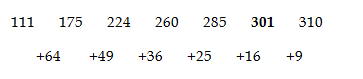# RRB ALP 2018 Practice Test Papers | Arithmetic Questions (Day-83)

Dear Aspirants, Here we have given the Important RRB ALP & Technicians Exam 2018 Practice Test Papers. Candidates those who are preparing for RRB ALP 2018 can practice these Arithmetic Questions to get more confidence to Crack RRB 2018 Examination.

[WpProQuiz 3178]

Click “Start Quiz” to attend these Questions and view Solutions

1) The side of a cube is 8 cm. Find the diagonal of the cube.

a) 6 √3

b) 7 √3

c) 8 √3

d) 9 √3

2) Ratio between the speeds of two cars is 5 : 7. If the fastest car runs 140 Km in 5 hours, then what is the speed of another car?

a) 10 kmph

b) 15 kmph

c) 20 kmph

d) 25 kmph

3) If 10 men can finish the work in 30 days, then how many days the work will be completed in 10 men with twice the efficiency?

a) 15 days

b) 7. 5 days

c) 10 days

d) 25 days

4) Find 2 sin x.cos x =

a) Cos 2x

b) Sin2x

c) 2.Sin2x

d) Sin2x .cos2x

5) Find the value of Cosec 90°+ sec 60°

a) 1

b) 0

c) 3

d) -1

6) If 30 % of A = 50 % of B, the what is the ratio of A:B?

a) 3 : 5

b) 5 : 3

c) 4: 5

d) 5: 4

7) Find the next of the give series

111, 175, 224, 260, 285, ? , 310

a) 300

b) 289

c) 301

d) 344

8) Average age of 5 girls and 5 boys is 20 years and 22 years respectively. Find the total average age of all the students.

a) 20

b) 22

c) 21 .5

d) 21

9) 440 + √50 ÷7 =?

a) 4

b) 7

c) 22

d) 21

10) (344 + 908 + 978 – 867 – 230 – 123) ÷ 4 = ?

a) 225.5

b) 221.5

c) 234.5

d) 252.5

Diagonal of a cube =√3a

a = 8

Diagonal of a cube =√3 (8)

Diagonal of a cube = 8√3

Speed of Fastest cars = 140 / 5 = 28 kmph

Speed of lowest car = (28*5)/7 = 20 kmph

10 men ——– 30 days

10 men ———  ?        Twice the efficiency

10 * 30 = 2 * 10 * x

X = 15 days

2 sin x.cos x = Sin2x

Cosec 90° + sec 60 ° = 1 + 2 = 3

30 A /100 = 50 B/100

A : B = 5 : 3Total age of girls = 20 * 5 = 100

Total age of boys = 22 * 5 = 110

Total Average = Total Age/Total No. of students

= (100 + 110)/ 10 = 210/10 = 21

√440 ≈ √ 441 = 21

√50 ≈ √49 = 7

√440 + √50 ÷7 = 21 + (7 ÷ 7) = 21+1 = 22

344 + 908 + 978 – 867 – 230 – 123
= 2230 – 1220
= 1010 ÷ 4
= 252. 5

RRB ALP 2018 – “All in One” Study Materials and Practice Sets